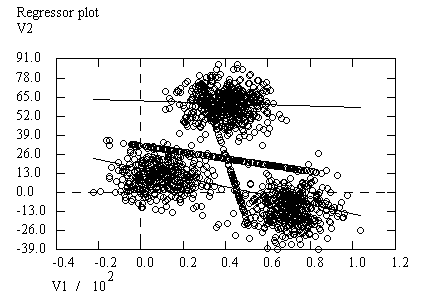Compartilhar:

#### MIXTURE REGRESSION MODEL FOR INCOMPLETE DATA

Loc Nguyen, Anum Shafiq

ABSTRACT
The Regression Expectation Maximization (REM) algorithm, which is a variant of Expectation Maximization (EM) algorithm, uses parallelly a long regression model and many short regression models to solve the problem of incomplete data. Experimental results proved resistance of REM to incomplete data, in which accuracy of REM decreases insignificantly when data sample is made sparse with loss ratios up to 80%. However, as traditional regression analysis methods, the accuracy of REM can be decreased if data varies complicatedly with many trends. In this research, we propose a so-called Mixture Regression Expectation Maximization (MREM) algorithm. MREM is the full combination of REM and mixture model in which we use two EM processes in the same loop. MREM uses the first EM process for exponential family of probability distributions to estimate missing values as REM does. Consequently, MREM uses the second EM process to estimate parameters as mixture model method does. The purpose of MREM is to take advantages of both REM and mixture model. Unfortunately, experimental result shows that MREM is less accurate than REM. However, MREM is essential because a different approach for mixture model can be referred by fusing linear equations of MREM into a unique curve equation.
Keywords: Regression Model, Mixture Regression Model, Expectation Maximization Algorithm, Incomplete Data

Compartilhar: